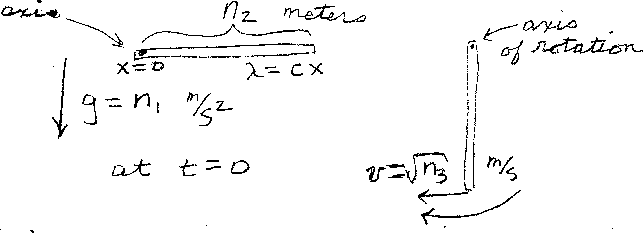Problem D11: A rod has a linear mass density of the form λ = cx, where x is the distance from the end and c is a constant. The rod can swing freely with one end fixed where x=0. It starts out horizontal at rest and is let go. The length of the rod is n2 meters, and the acceleration due to gravity is n1 m/s2. What is the speed of the tip of the rod as it swings through the bottom? If the speed of the rod is v=√ n3 m/s. What is n3? Note that the units of n3 are m2/sec2.n1 = n2 = Input n3:
If you are currently in my class, you can record your grade by entering your name and student ID number (without the leading zeros) below and clicking on "record grade".
 First Name = Last Name = ID = Problem: